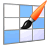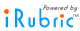# iRubric: 2 digit Multiplication rubric

 find rubric
 delete .rubinfoitem { padding:5px; white-space: nowrap; } 2 digit Multiplication  5.NBT.B.5 Rubric Code: C237X7X By jaymil18 Ready to use Public Rubric Subject: Math   Type: Assessment   Grade Levels: K-52 digit multiplication 5.NBT.B.5 Poor 1 pts Fair 2 pts Good 3 pts Excellent 4 pts Comments (N/A) Student can solve addition and multiplication 2 pts Student is able to fluently add and multiply multi digit numbers to solve an equation. Poor Student is unable to add or multiply single digit or multi-digit numbers to solve an equation. Fair Student is able to add and multiply single digit numbers but needs help when adding or multiplying multi-digit numbers to solve equations. Good Student is able to add and multiply single and multi-digit numbers to solve equation. Excellent Student is able to add and multiply single digit and multi-digit numbers. Student is able to verbally explain the process of how they solved the equation Comments Student is able to set up equation 3 pts Student should be able to set up an equation using 2 digit numbers and understands the place value of the multi-digit whole numbers Poor Student is unable to line up numbers to multiply and can not explain the place values in a 2 digit number Fair Student is able to line up the numbers correctly but can not explain the place values in a 2 digit number. Good Student is able to line up the numbers correctly and understands the place values of a 2 digit number. Excellent Student is able to line up the numbers to set up the equation and understands the place values of a 2 digit number. Student is able to explain the equation to others. Comments Student is able to solve the equation 2 pts Student is able to solve a multi-digit whole number equation using different strategies. Poor Student is unable to use one strategy like standard algorithm to solve the equation. Fair Student is able to solve using one strategy like standard algorithm but needs guidance when using a different approach to solving the equation. Good Student is able to solve multi-digit equations using multiple strategies and is able to explain which strategy is the most efficient to use. Student can explain why the strategy works using place value or order of operations. Excellent Student is able to solve multi-digit equations using multiple strategies and is able to explain which strategy is the most efficient for them. Student can explain why the strategy works using place value or order of operations. Student as well can apply the knowledge to real world situations. Comments Student can solve a word problem 4 pts Student is able to read a word problem and use the information to set up a multi-digit equation and solve. Student uses a label correctly when giving the solution to the equation Poor Student is unable to read a word problem and pick out the numbers for the equation. Student is unable to set up and solve equation without assistance. Student is unable to provide the solutions label Fair Student is able to read a word problem and pick out the important information. Student needs assistance when setting up the equation and solving the equation. Student is able to give a label. Good Student is able to read a word problem and pick out the important information. Student can independently set up the equation and solve the equation. Student is able to give correct solution and label correctly. Excellent Student is able to read a word problem and pick out the important information. Student can independently set up the equation and solve the equation. Student is able to give correct solution and label correctly. Student is able to explain why they set up the equation the way they did and explain why a label is needed. Comments Comments 0 pts Poor Fair Good Excellent Comments Score 2 pts Total Score______/52 Poor Fair Good Excellent Comments

Subjects:

Types:

You may also be interested in:

Do more with this rubric:

Preview this rubric.

Modify this rubric.

Make a copy of this rubric and begin editing the copy.

Show a printable version of this rubric.

Add this rubric to multiple categories.

Bookmark this rubric for future reference.
Assess

Test this rubric or perform an ad-hoc assessment.

Build a gradebook to assess students.

Apply this rubric to any object and invite others to assess.
Share

Email this rubric to a friend.

Discuss this rubric with other members.

Do more with rubrics than ever imagined possible.

Only with iRubrictm.